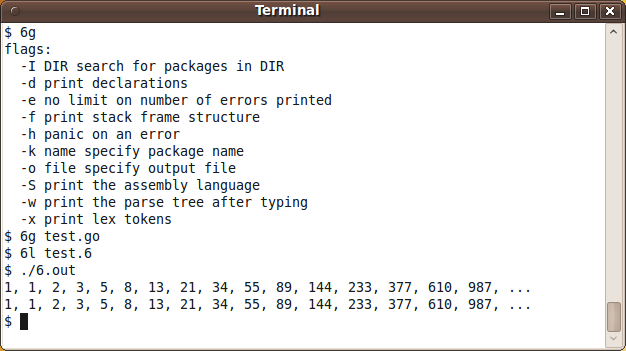# gc (8g, 6g)

Implementation of programming language Go

gc is written in C using yacc/bison for the parser. 6g is the Go compiler for amd64, and 8g — for 386.Go's gc compiler screenshot

## Examples:

### Fibonacci numbers:

Example for versions gc-2010-07-14

This example implements all three methods of calculating Fibonacci numbers — recursive, iterative and Binet’s formula. Besides, a generic function for printing results of using any of the functions is defined.

``````package main
import ("fmt"
"math")

//Fibonacci Recursive
func fibr(n int) int {
if n < 2 { return 1 }
return fibr(n-2) + fibr(n-1)
}

//Fibonacci Iterative
func fibi(n int) int {
var a, b int = 1, 1
for i := 0; i < n; i++ {
a, b = b, a+b
}
return a
}

//Fibonacci Binet
func fibb(n int) int {
g := (1 + math.Sqrt(5)) / 2
ret := (math.Pow(g, float64(n)) - math.Pow(1-g, float64(n))) / math.Sqrt(5)
return int(ret)
}

type fibfunc func(int) int

//Implements a general printing method for fibonacci functions
func printFib(fib fibfunc, a, b int) {
for i := a; i < b; i++ {
fmt.Printf("%d, ", fib(i))
}
fmt.Println("...")
}

func main() {
printFib(fibr, 0, 16)
printFib(fibi, 0, 16)
printFib(fibb, 1, 17)
}
``````

### Factorial:

Example for versions gc-2010-07-14

The example implements both recursive and iterative methods of calculating factorial and a generic function for printing the results of using any of the methods.

``````package main
import "fmt"

//Recursive Factorial
func factr(n uint64) uint64 {
if (n < 2) { return 1 }
return n * factr(n-1)
}

//Iterative Factorial
func facti(n uint64) uint64 {
var ret uint64 = 1
for ; n > 0; n-- {
ret *= n
}
return ret
}

func printFact(fact func(uint64) uint64) {
for i := 0; i < 17; i++ {
fmt.Printf("%d! = %d\n", i, fact(uint64(i)))
}
}

func main() {
printFact(factr)
fmt.Println("--")
printFact(facti)
}
``````

### Hello, World!:

Example for versions gc-2010-07-14
``````package main
import "fmt"

func main() {
fmt.Println("Hello, World!")
}
``````

Example for versions gc-2010-07-14

When you import several packages, you can put a dot before name of one of them, and use its functions without a package-name prefix, like `fmt` in this example.

To read a number, one has to read a string from standard input, remove the trailing ‘n’ and convert it into the wanted format. In our case, input variables are integer, but further on we’ll be using them for floating-point calculations. Go doesn’t support implicit type conversions, so it’s better to parse input as float64 (the type of argument for `math.Sqrt`) right away.

Assignment = is plain one, while := means that the variable on the left side is defined in this line. Note that defined but unused variables count as compilation errors, so you have to use `err` in some way.

``````package main
import (
"os"
. "fmt"
"math"
"bufio"
"strconv")

func main() {
line = line[0 : len(line)-1]
A, err := strconv.Atof64(line)
if (A == 0) {
return
}
line = line[0 : len(line)-1]
B, err := strconv.Atof64(line)
line = line[0 : len(line)-1]
C, err := strconv.Atof64(line)
if err != nil {
Print(err)
}
D := B*B-4*A*C
if (D == 0) {
Printf("x = %f", -B/2/A)
return
}
if (D>0) {
Printf("x1 = %f\nx2 = %f", -B/2/A + math.Sqrt(D)/2/A, -B/2/A - math.Sqrt(D)/2/A)
} else {
Printf("x1 = (%f, %f)\nx2 = (%f, %f)", -B/2/A, math.Sqrt(-D)/2/A, -B/2/A, -math.Sqrt(-D)/2/A)
}
}
``````